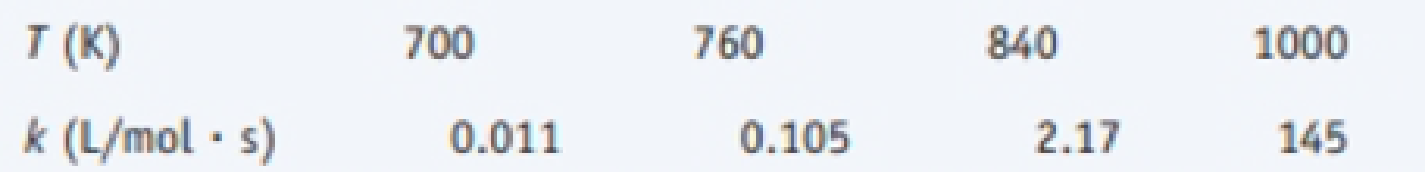Chapter 14.5, Problem 14.10CYU

Chapter
Section
Textbook Problem

Rate constants were determined for the decomposition of acetaldehyde (CH3CHO) in the temperature range 700 to 1000 K. Use these data to determine Ea for the reaction using a graphical method.Interpretation Introduction

Interpretation: The value of activation energy has to be determined.

Concept introduction:

Arrhenius equation:

Arrhenius equation is used to calculate the rate constant of many reactions. Arrhenius equation takes the form

k=Ae-Ea/RT

Where,

k=rate constant

A=frequency factor

e=base of logarithms

Ea = energy of activation

T=Temperature

Mathematically, the above equation can be written as,

lnk=lnA-EaRT

Determination of activation energy: If the rate constant for a given reaction are measured at several temperatures, graphical techniques used to determine the activation energy of a reaction.

lnk =-(EaR)1T+lnA Equation for straight line:_lnkindicates y-axis.(EaR)indicates slope1T indicates x-axislnAindicates intercept.

Explanation

The activation energy is calculated as,

Experiment1/T(K1)lnk10.0014294.509920.0013162.253830.0011900.774740.0014.9767slope:=(1

Still sussing out bartleby?

Check out a sample textbook solution.

See a sample solution

The Solution to Your Study Problems

Bartleby provides explanations to thousands of textbook problems written by our experts, many with advanced degrees!

Get Started

Find more solutions based on key concepts